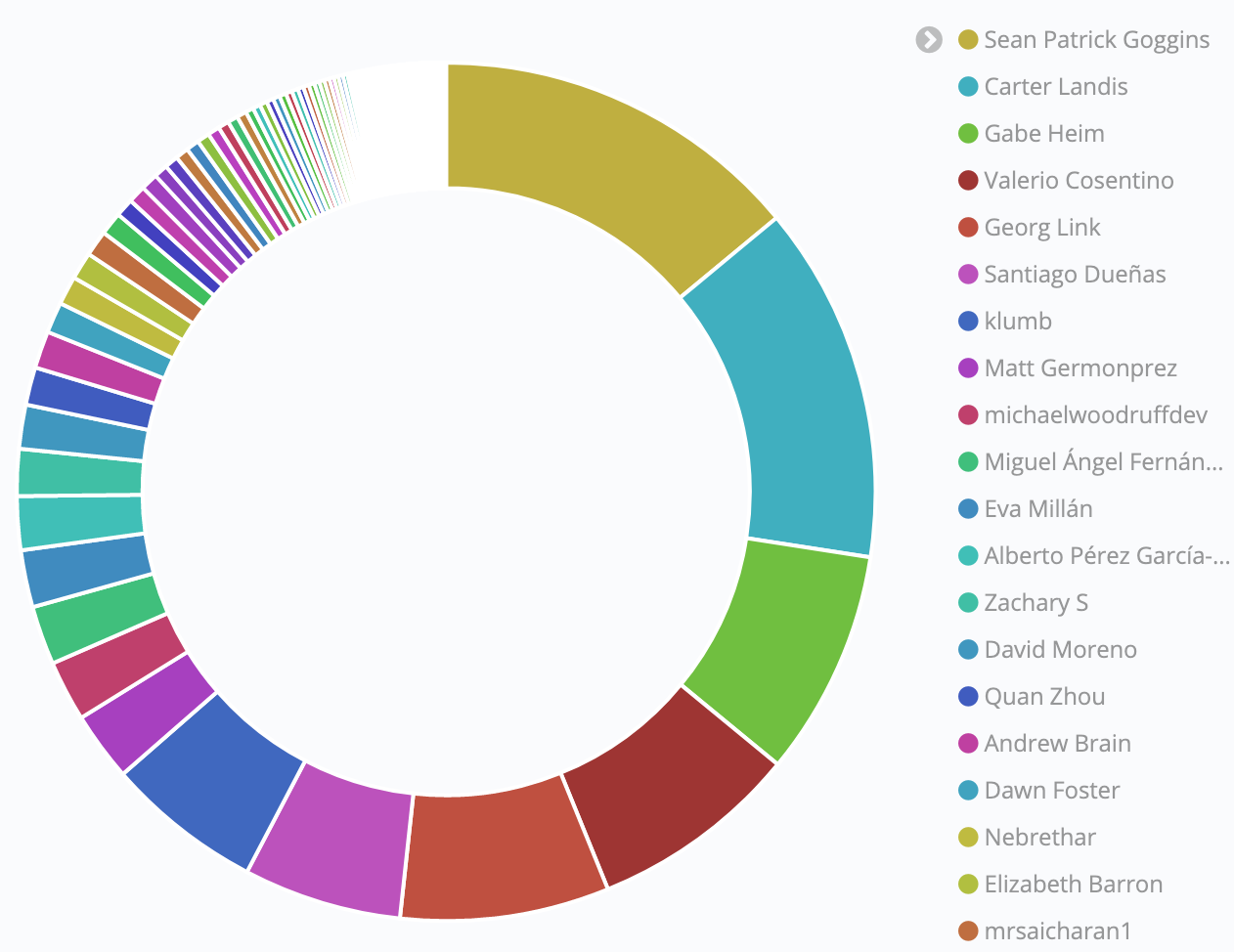# Bus Factor

Question: How high is the risk to a project should the most active people leave?

## Description

The Bus Factor is a compelling metric because it visualizes the question "how many contributors can we lose before a project stalls?" by hypothetically having these people get run over by a bus (more pleasantly, how many would have to win in a lottery and decide to move on).

The Bus Factor is the smallest number of people that make 50% of contributions.

## Objectives

• Identify how widely the work in a project is distributed across contributors.
• Identify the key people in a project that are doing the majority of the work.

## Implementation

The formula for Bus Factor is a percentage calculation -50% will be our threshold- followed by adding up each contributor's contributions sorted in decreasing order until we reach the threshold.

If we have 8 contributors who each contribute the following number of contributions to a project: `1000, 202, 90, 33, 332, 343, 42, 433`, then we can determine the Bus Factor by first identifying the 50% of total contributions for all the contributors.

Summary: 50% of total contributions = `1,237.5`, so the Bus Factor is `2`.

Full Solution:

1. Arrange the data in descending order: `1000, 433, 343, 332, 202, 90, 42, 33`
2. Compute the 50% of the total:
• `(1,000 + 433 + 343 + 332 + 202 + 90 + 42 + 33) * 0.5 = 1,237.5`
3. Adding up the first two contributors in our ranking we get `1,433`.
4. Answer: as `1,433 > 1,237.5`, more than the 50% of contributions is performed by only `2` contributors, thus the `Bus Factor = 2`.

### Filters

• Time: The Bus Factor may be vary for different time periods. The Bus Factor over the life of a project may misrepresent the current level of contributor engagement in the project.
• Repository Group: Many open source projects include multiple repositories, and in some cases examining all of the repositories associated with any given project provides a more complete picture of the Bus Factor.

### Visualizations (optional)The Bus Factor for the CHAOSS Project in 2020, when considering only git commits, was 5.

### Tools Providing the Metric

1. Augur
2. GrimoireLab provides this metric out of the box, not as a single number but as a visualization.

### Data Collection Strategies

The data collection strategy depends on the Types of Contributions used for calculating the Bus Factor. Bus Factor is often calculated for commits but other types of contributions can be used for this as well, separately or combined.